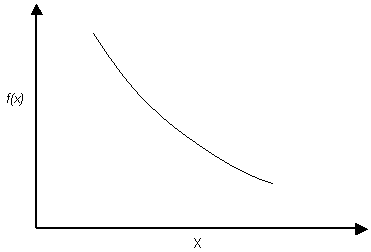The Rocscience International Conference 2021 Proceedings are now available. Read Now

# Exponential Distribution

The EXPONENTIAL distribution is available in RocSupport for modelling input variables. The following figure shows a typical exponential probability density function.

Exponential probability density functionNOTES:

1. The range of values must always be positive for an EXPONENTIAL distribution.
2. The mean of an EXPONENTIAL distribution is equal to its standard deviation. This is a property of the EXPONENTIAL distribution, and cannot be altered by the user. (This is why you cannot define a standard deviation for an EXPONENTIAL distribution in RocSupport).
3. Like the NORMAL distribution, the EXPONENTIAL distribution can be truncated by entering the desired minimum and maximum values (the basic EXPONENTIAL distribution varies from zero to infinity).